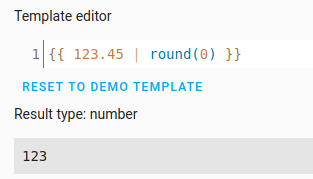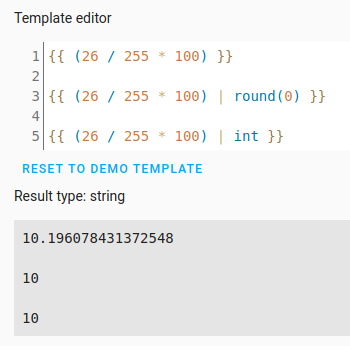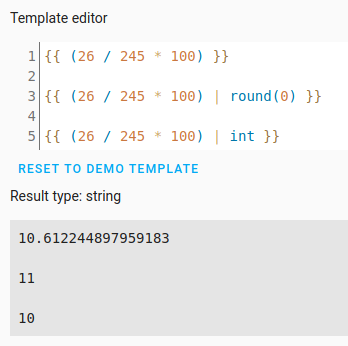# Jinja math problem, HA isn't rounding or converting to a int

I’m trying to use the Live Sensor Report Example Automation provided in the Alexa Media Player github wiki.

What I have
The math should use the ‘brightness’ attribute from a light.alexa_virtual and convert it in to a percent integer.

``````{{ state_attr('light.alexa_virtual', 'brightness') | int / 255 * 100 | int }}
``````

The above is the math part of the conditional:

``````{% if (state_attr('light.alexa_virtual', 'brightness') | int / 255 * 100 ) | int  == 1  %}
``````
Note

Note: the given example uses `states.light.alexa_virtual.attributes.brightness ` but I changed it to `state_attr('light.alexa_virtual', 'brightness')`.

The math
If `state_attr('light.alexa_virtual', 'brightness')` = 26
then the result should be 10 right?

`````` {{ state_attr('light.alexa_virtual', 'brightness') | int / 255 * 100 | int }}
{{ 26                                              | int / 255 * 100 | int }}
``````

The result should be 10 but when I put it in the Developer Tools > Template editor it shows 10.196078431372548.

What I’ve tried
I’ve tried using `round` `{{ 26 | int / 255 * 100 | round | int }}` but that didn’t change anything.

What am I doing wrong?

INT and round only works on the object to the left, so you should try to used more brackets as in your example you rounded 100 and made in INT

`{{ (26 | int / 255 * 100) | round(0) | int }}`

Or try something like ` {{ (( float / 255 ) * 100) | int }}`

Thank you for explaining that. Adding `round(0)` fixes it. I’ll send a correction to the yaml to the dev to update their wiki.

Here is the final corrected condition:

``````{% if (state_attr('light.alexa_virtual', 'brightness') | int / 255 * 100 ) | round(0) | int  == 1  %}
``````

Side question for understanding…
Does `| int` round using floor?

When I have `state_attr('light.alexa_virtual', 'brightness')` = 10 without `round(0)` it comes out to 3, but when I add `| round(0)` it comes out to the expected 4.

`{{ 26 | int / 255 * 100 | int }}` = 3 <- Incorrect
`{{ 26 | int / 255 * 100 | round(0) | int }}` = 4 <- Correct

What does `| int` do that does not have the expected behavior?

It is Python math that is used, so
`Int` will round down (floor) to nearest integear, while `round(0)` will round to nearest integear.

Why have you included the `int` filter after using `round(0)` in your template?

``````| round(0) | int
``````

from here:

``````{% if (state_attr('light.alexa_virtual', 'brightness') | int / 255 * 100 ) | round(0) | int  == 1  %}
``````

Using `round(0)` will round the value to an integer so running it through the `int` filter is redundant.For that matter, the template in the Solution post uses `int` unnecessarily twice.

``````{{ (26 | int / 255 * 100) | round(0) | int }}
^                             ^
|                             |
Not needed
``````Another example:Hi, what i doing wrong here ? i need intiger ?

Copy-paste this into the Template Editor and it will report it is a number and the value will not have a any decimal portion (it will be an integer):

``````{{ (state_attr('climate.hmip_etrv_2_000a1d8997d5e7', 'level') | float(0) * 100) | round(0) | int(0) }}
``````

If you add double-quotes outside of the template, the Template Editor will report it is a string. However, that’s just the way the Template Editor works and doesn’t mean the template’s result will be handled as a string when the template is used elsewhere.

1 Like